# Year 2 Maths Printable Worksheets

Use our colourful year 2 maths worksheets to help your child master new maths skills! Our maths worksheets cover all the core objectives including problem-solving, shape and space, mental maths, number bonds, and measuring and recording results using tables. We also cover topics such as money, fractions, greater than/less than, ordering and comparing, and more.

Filters

### Divide by 5 spider web

Year 2 Maths - This fun worksheet will help year 2 pupils practice dividing numbers by 5. Look at the numbers in the spider web and divide each number by 5. Then complete the sums at the bottom of the page.

Age: 6 - 7
1
2
3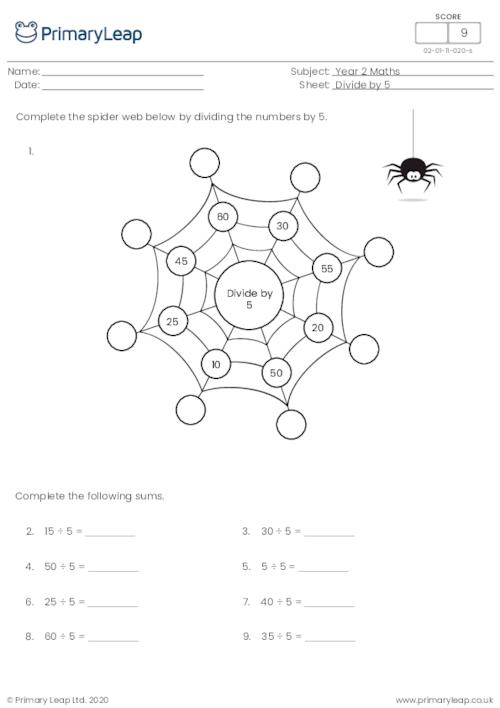### Bar Modelling - 10 xtable

Bar models can be used to solve different kinds of multiplication. Students answer these 10x table questions as quickly as possible.

Age: 6 - 7
1
2
3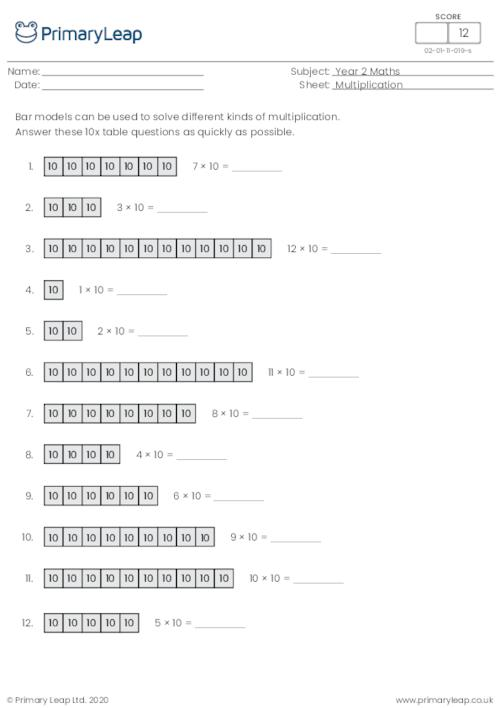### Bar Modelling - 5 xtable

Bar models can be used to solve different kinds of multiplication. Students answer these 5x table questions as quickly as possible.

Age: 6 - 7
1
2
3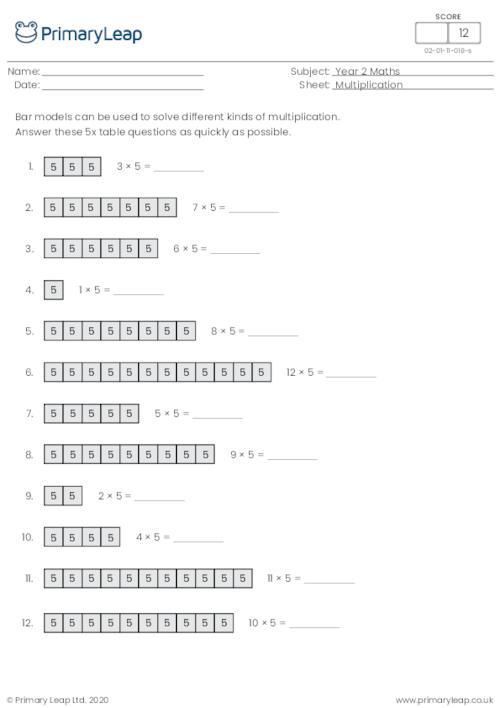### Bar Modelling - 2 xtable

Bar models can be used to solve different kinds of multiplication. Answer these 2x table questions as quickly as possible.

Age: 6 - 7
1
2
3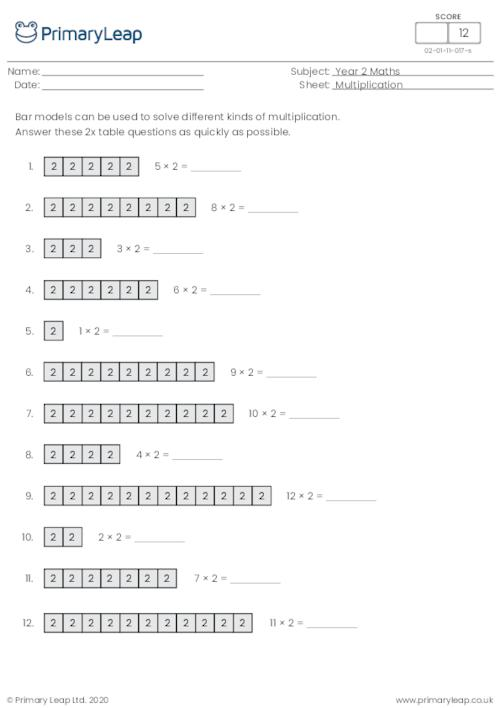### Divide by 2 Spider's Web

Year 2 Maths - This fun worksheet will help year 2 pupils practice dividing numbers by 2. Look at the numbers in the spider's web and divide each number by 2. Then complete the sums at the bottom of the page.

Age: 6 - 7
1
2
3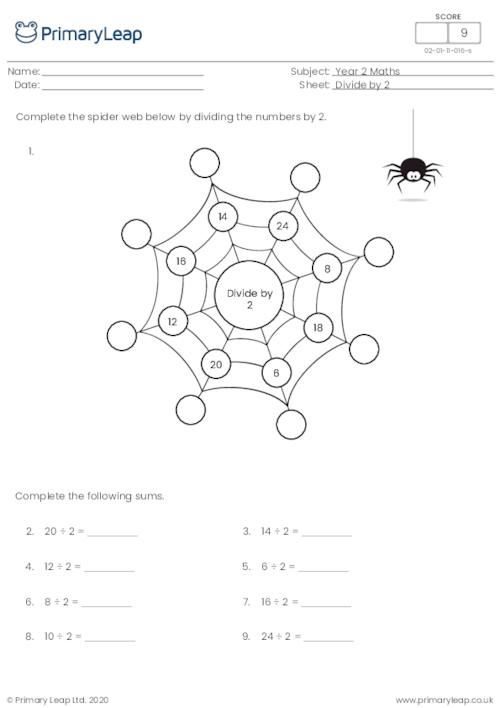### Multiply, divide, subtract or add by 4 and 10

Year 2 maths - Multiply, divide, subtract or add worksheet. This activity asks children to look at the sums and fill in the correct sign to make the sums correct. The sums include the numbers 4 and 10.

Age: 6 - 7
1
2
3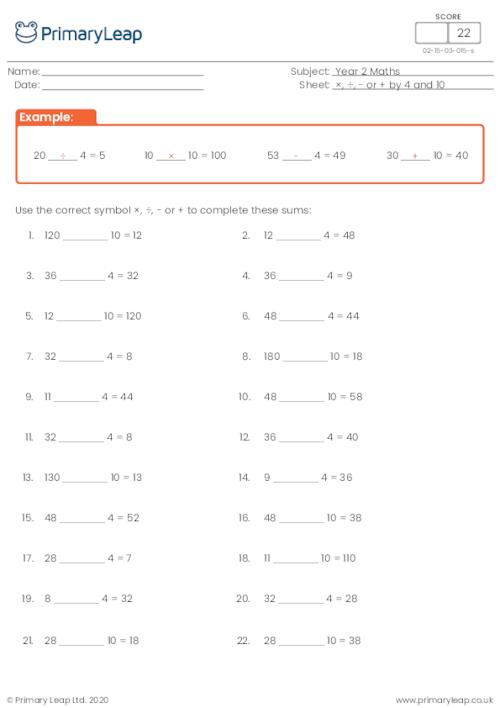### Multiply, divide, subtract or add by 4 and 10

Year 2 maths - Multiply, divide, subtract or add worksheet. This activity asks children to look at the sums and fill in the correct sign to make the sums correct. The sums include the numbers 4 and 10.

Age: 6 - 7
1
2
3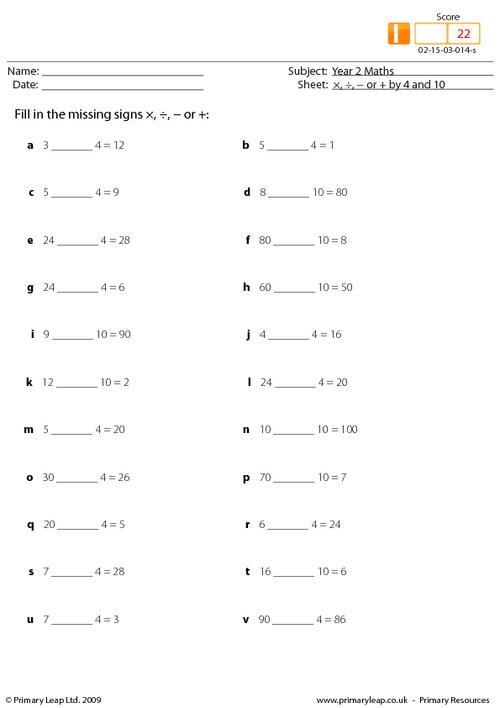### Multiply, divide, subtract or add by 4 and 10

Year 2 maths - Multiply, divide, subtract or add worksheet. This activity asks children to look at the sums and fill in the correct sign to make the sums correct. The sums include the numbers 4 and 10.

Age: 6 - 7
1
2
3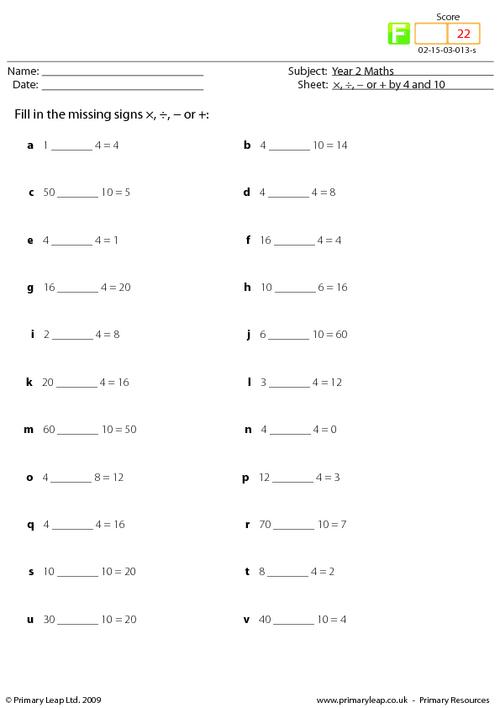### Multiply or divide

Year 2 maths - Multiply or divide worksheet. This activity asks children to look at the sums and fill in the correct sign to make the sums correct.

Age: 6 - 7
1
2
3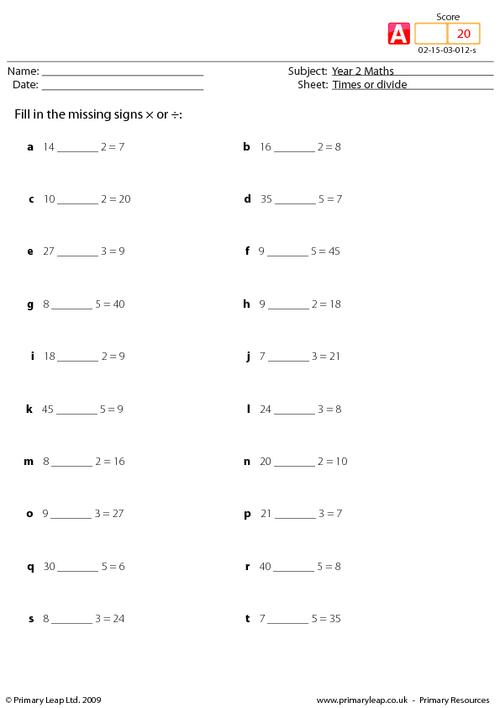### Multiply or divide

Year 2 maths - Multiply or divide worksheet. This activity asks children to look at the sums and fill in the correct sign to make the sums correct.

Age: 6 - 7
1
2
3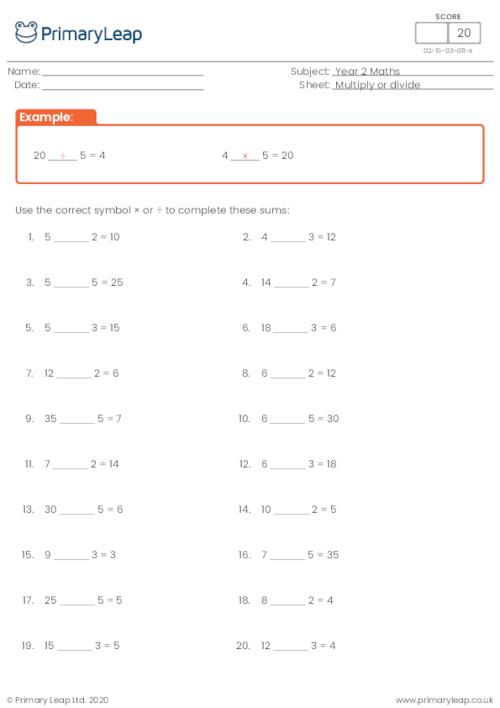### Multiply or divide

Year 2 maths - Multiply or divide worksheet. This activity asks children to look at the sums and fill in the correct sign to make the sums correct.

Age: 6 - 7
1
2
3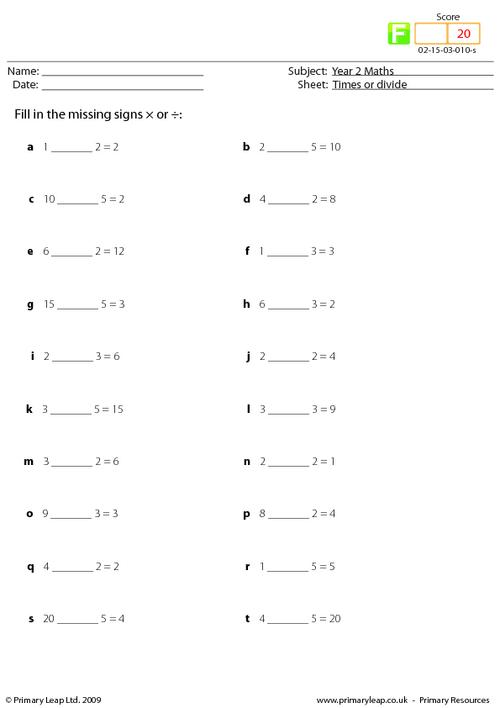### Divide by 2, 3 and 5 mixed

Year 2 maths - Division worksheet. Division is the process by which we try to find out how many times a number (divisor) will go into another number (dividend). This page includes 20 sums dividing by 2, 3 and 5.

Age: 6 - 7
1
2
3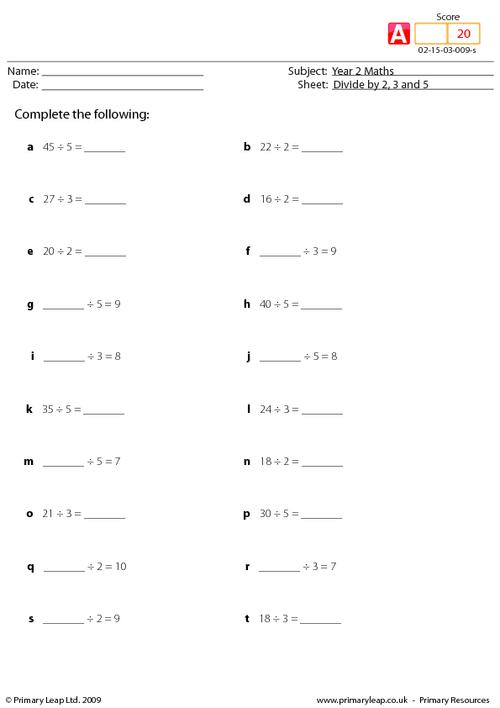### Divide by 2, 3 and 5 mixed

Year 2 maths - Division worksheet. Division is the process by which we try to find out how many times a number (divisor) will go into another number (dividend). This page includes 16 sums dividing by 2, 3 and 5.

Age: 6 - 7
1
2
3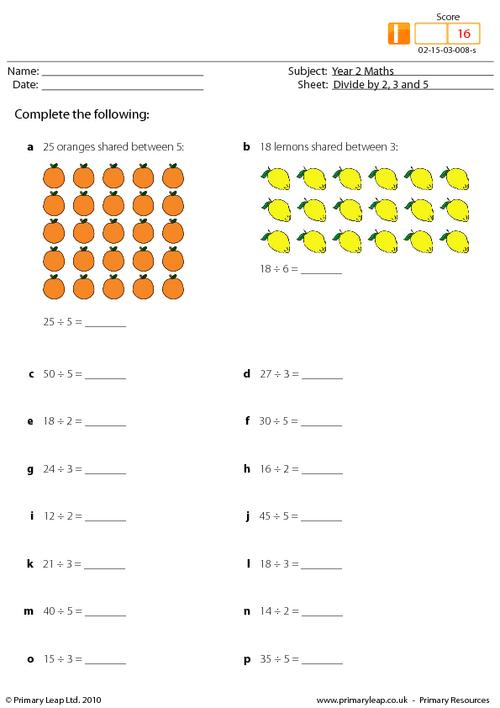### Divide by 2, 3 and 5 mixed

Year 2 maths - Division worksheet. Division is the process by which we try to find out how many times a number (divisor) will go into another number (dividend). This page includes 16 sums dividing by 2, 3 and 5.

Age: 6 - 7
1
2
3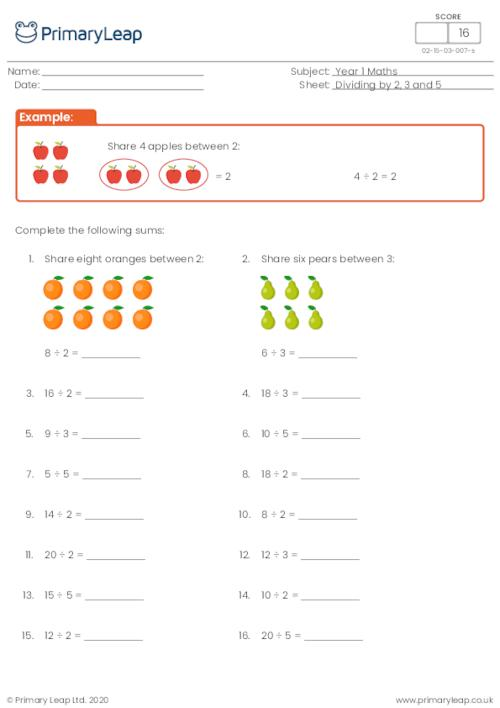### Multiply by 3 and 4 mixed

Year 2 maths - Multiplication worksheet. This primary activity covers the three and four times table. Having a good understanding of times tables will help because future maths skills build on this knowledge, and without it pupils will struggle later on.

Age: 6 - 7
1
2
3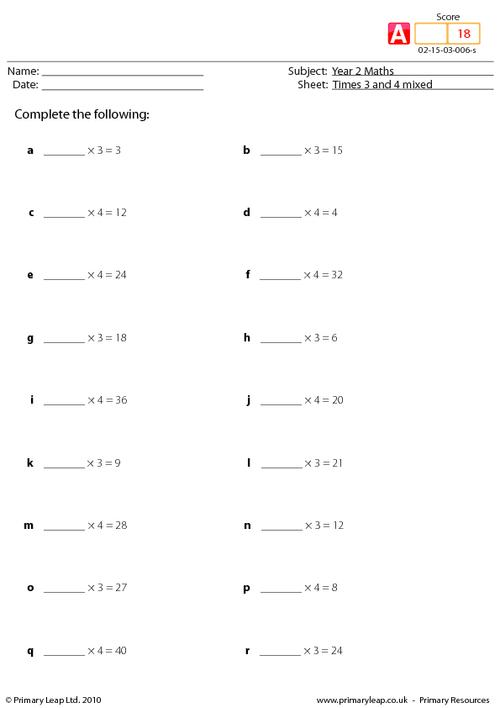### Multiply by 3 and 4 mixed

Year 2 maths - Multiplication worksheet. This primary activity covers the three and four times table. Having a good understanding of times tables will help because future maths skills build on this knowledge, and without it pupils will struggle later on.

Age: 6 - 7
1
2
3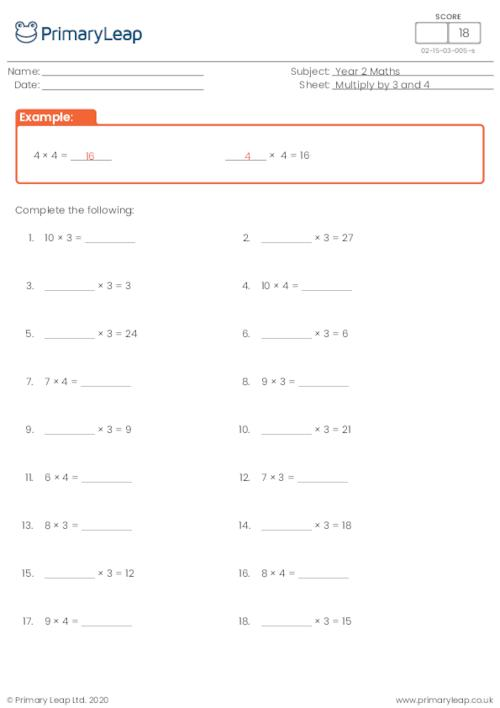### Multiply by 3 and 4 mixed

Year 2 maths - Multiplication worksheet. This primary activity covers the three and four times table. Having a good understanding of times tables will help because future maths skills build on this knowledge, and without it pupils will struggle later on.

Age: 6 - 7
1
2
3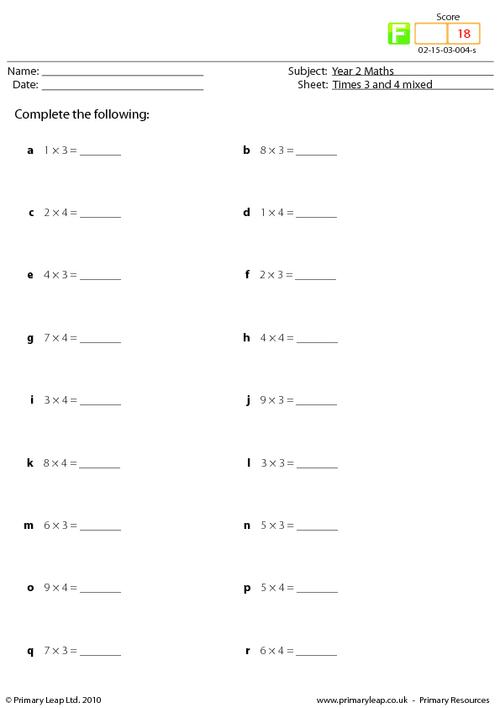### Multiply by 2, 5 and 10 mixed

Year 2 maths - Multiplication worksheet. This primary activity covers the two, five and ten times table. Having a good understanding of times tables will help because future maths skills build on this knowledge, and without it pupils will struggle later on.

Age: 6 - 7
1
2
3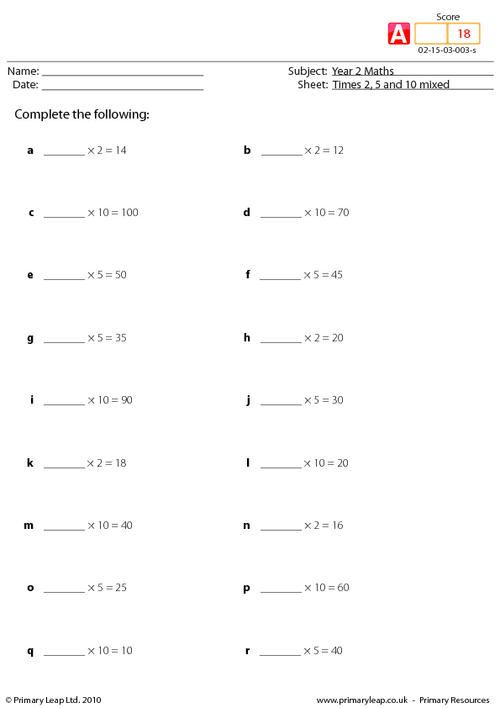### Multiply by 2, 5 and 10 mixed

Year 2 maths - Multiplication worksheet. This primary activity covers the two, five and ten times table. Having a good understanding of times tables will help because future maths skills build on this knowledge, and without it pupils will struggle later on.

Age: 6 - 7
1
2
3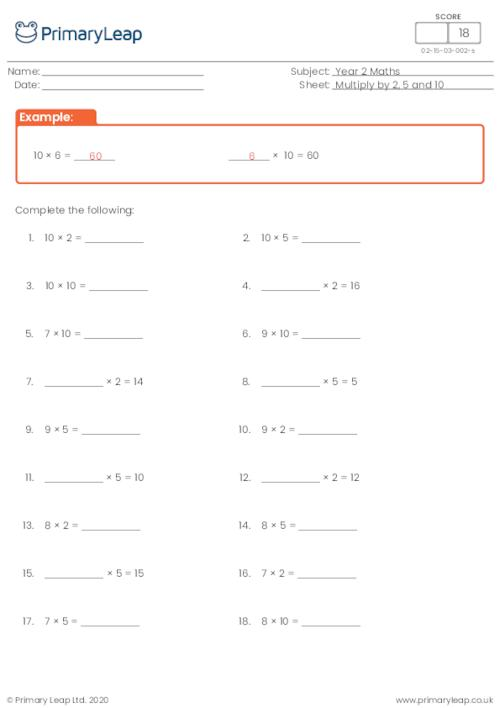### Multiply by 2, 5 and 10 mixed

Year 2 maths - Multiplication worksheet. This primary activity covers the two, five and ten times table. Having a good understanding of times tables will help because future maths skills build on this knowledge, and without it pupils will struggle later on.

Age: 6 - 7
1
2
3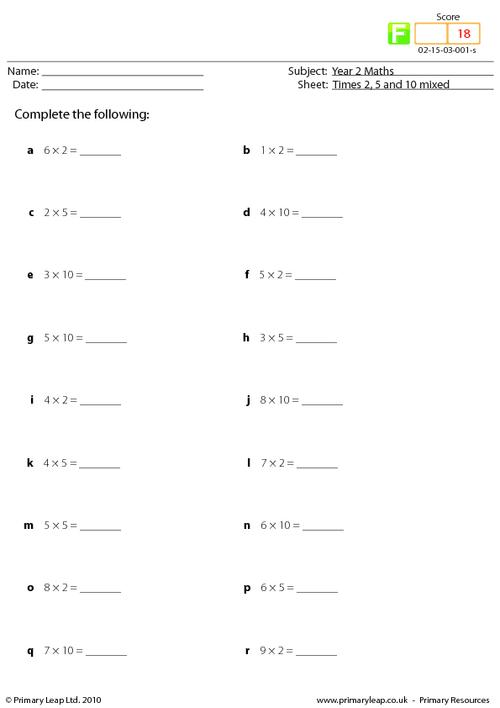of 1Help your child find the joy of learning year 2 maths with our engaging maths activities. We have hundreds of year 2 maths worksheets to boost your child's confidence. Our maths worksheets will help your child learn how to count in steps of 2, 3, 5 and 10, learn number bonds to 20, add and subtract one-digit and two-digit numbers, find fractions of shapes, and more.Learn

One of the best things about printable worksheets is that they make learning fun and easy. Explore our educational resources based on Year 2 Maths and keep your children learning through these fun and engaging exercises.Progress

Our Year 2 Maths printable worksheets are designed for progressive learning. Our engaging content enables a child to learn at their own speed and gain confidence quickly. When we help a child believe in themselves, we empower them for life.Improve

Printable worksheets are a great way to enhance creativity and improve a child’s knowledge. Help your child learn more about Year 2 Maths with this great selection of worksheets designed to help children succeed.